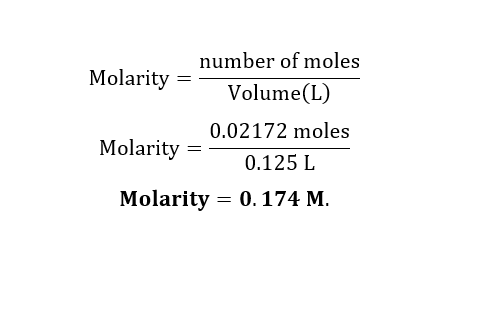# Consider the neutralization reaction2HNO3(aq)+Ba(OH)2(aq)⟶2H2O(l)+Ba(NO3)2(aq)2HNO3(aq)+Ba(OH)2(aq)⟶2H2O(l)+Ba(NO3)2(aq)A 0.125 L0.125 L sample of an unknown HNO3HNO3 solution required 54.3 mL54.3 mL of 0.200 M Ba(OH)20.200 M Ba(OH)2 for complete neutralization. What is the concentration of the HNO3HNO3 solution?

Question
56 views

Consider the neutralization reaction

2HNO3(aq)+Ba(OH)2(aq)⟶2H2O(l)+Ba(NO3)2(aq)2HNO3(aq)+Ba(OH)2(aq)⟶2H2O(l)+Ba(NO3)2(aq)

A 0.125 L0.125 L sample of an unknown HNO3HNO3 solution required 54.3 mL54.3 mL of 0.200 M Ba(OH)20.200 M Ba(OH)2 for complete neutralization. What is the concentration of the HNO3HNO3 solution?

check_circle

Step 1

Given:

Volume of HNO3 = 0.125 L

Volume of Ba(OH)2 = 54.3 mL = 0.0543 L.

Concentration of Ba(OH)2 = 0.200 M

Chemical reaction is given by,

2HNO3(aq) + Ba(OH)2(aq)⟶ 2H2O(l) + Ba(NO3)2(aq)

Step 2

Calculation for moles of Ba(OH)2(aq):Step 3

Calculation for Concentration of HNO3:

2 moles HNO3 of  reacts with 1 mole of Ba(OH)2.

0.02172 moles HNO3 of  reacts with 0.01086 mole of Ba(OH)2....

### Want to see the full answer?

See Solution

#### Want to see this answer and more?

Solutions are written by subject experts who are available 24/7. Questions are typically answered within 1 hour.*

See Solution
*Response times may vary by subject and question.
Tagged in

### Chemistry